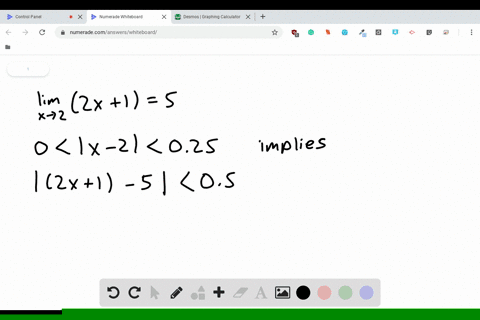Enroll in one of our FREE online STEM bootcamps. Join today and start acing your classes!View Bootcamps01:00
Problem 2

# Limits That Fail to Exist Identify three types of behavior associated with the nonexistence of a limit.Illustrate each type with a graph of a function.

## Discussion

You must be signed in to discuss.

## Video Transcript

and this problem were asked to name three types of behavior associated with the non existence of a limit. Then we have to demonstrate each type with the graphical. Exactly. So we're going to use one graph that shares all three examples. So we can kind of break this graph into through some photographs, if you like, but it kind of just put them on one grid. But we'll look at each type of example that we see here. So if we look right here at the behavior around negative too. Yeah, we see that to the left of negative, too. FX is approaching negative infinity. But as X is approaching negative too. From the great hand side after Lex is approaching positive infinity. So I will say we'll name this as our first type of example. For example, one we have that the left and right limits our infinite and do not agree. So this is kind of horn. You'll see some sort of for a glass of Tokay. That's usually the case. No. If we look over at the behavior around zero help, we see that the limit is approaches. Zero from the left appears to be 0.5, but from the right, it appears to be positive for, so the limit does not exist there. So this is an example of a case where the left and right limits are finite and they do not. They're finite, but they do not drink. Now. Finally, let's find our last example. So if you look over at the behavior of four, this one's interesting because we look at the left and right limits. They're the same. They're both approaching positive affinity. But positive affinity is not finite row value. So in this case, this is an example where the left right moments, I agree, but they are infinite values. So this is the's air. Three different examples of three types of behavior associated with the non existence of limits.